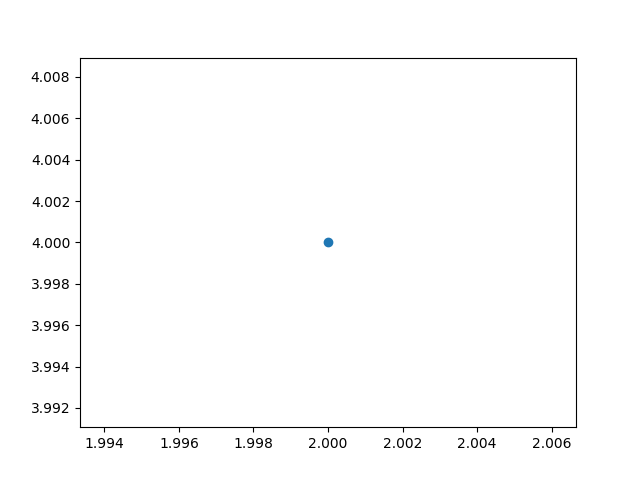﻿ python学习之matplotlib绘制散点图实例_python_脚本之家
python# python学习之matplotlib绘制散点图实例

```"""使用scatter()绘制散点图"""
import matplotlib.pyplot as plt

plt.scatter(2, 4)
plt.show()``````"""使用scatter()绘制散点图"""
import matplotlib.pyplot as plt

x_values = range(1, 6)
y_values = [x*x for x in x_values]
'''
scatter()
x:横坐标 y:纵坐标 s:点的尺寸
'''
plt.scatter(x_values, y_values, s=50)

# 设置图表标题并给坐标轴加上标签
plt.title('Square Numbers', fontsize=24)
plt.xlabel('Value', fontsize=14)
plt.ylabel('Square of Value', fontsize=14)

# 设置刻度标记的大小
plt.tick_params(axis='both', which='major', labelsize=14)
plt.show()``````"""使用scatter()绘制散点图"""
import matplotlib.pyplot as plt

x_values = range(1, 1001)
y_values = [x*x for x in x_values]
'''
scatter()
x:横坐标 y:纵坐标 s:点的尺寸
'''
plt.scatter(x_values, y_values, s=10)

# 设置图表标题并给坐标轴加上标签
plt.title('Square Numbers', fontsize=24)
plt.xlabel('Value', fontsize=14)
plt.ylabel('Square of Value', fontsize=14)

# 设置刻度标记的大小
plt.tick_params(axis='both', which='major', labelsize=14)

# 设置每个坐标轴的取值范围
plt.axis([0, 1100, 0, 1100000])
plt.show()``````"""使用scatter()绘制散点图"""
import matplotlib.pyplot as plt

x_values = range(1, 1001)
y_values = [x*x for x in x_values]
'''
scatter()
x:横坐标 y:纵坐标 s:点的尺寸
'''
plt.scatter(x_values, y_values, c=y_values, cmap=plt.cm.Blues, edgecolors='none', s=10)

# 设置图表标题并给坐标轴加上标签
plt.title('Square Numbers', fontsize=24)
plt.xlabel('Value', fontsize=14)
plt.ylabel('Square of Value', fontsize=14)

# 设置刻度标记的大小
plt.tick_params(axis='both', which='major', labelsize=14)

# 设置每个坐标轴的取值范围
plt.axis([0, 1100, 0, 1100000])
plt.show()```